### Sharing Ilmu

Sharing for useful things

### How To Configure OSPF LoadBalancing on Mikrotik

In this tutorial I’ll discuss about how to configuration of OSPF LoadBalancing. is case will be use when you have more than one network path that running ospf and you want to doing loadbalancing.

There is the topologi that are use for this tutorial :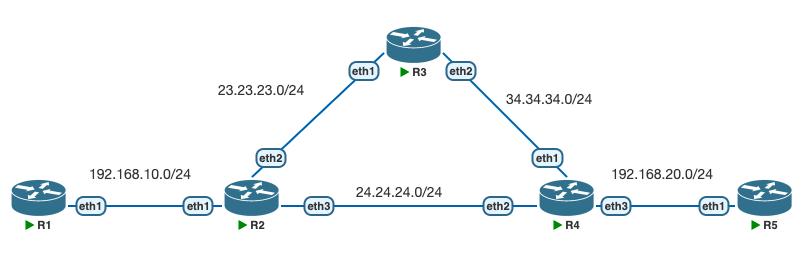As you know selection ospf best path with cost value and as the shown topologi IF R1 want connect to R5, route line will from R2 – R4 – R5. Because if you calculate the cost value, R2 – R4 has a cost value of 20 and R2 – R3 – R4 has a cost value of 30. Therefore best path network is R2 – R4.  and we want to do loadbalancing network so that if R1 want connect to R5 that can be connect from R2-R4 and R2-R3-R4.

Fist, doing basic configuration for each routers

R1

/system identity set name=R1

R5

/system identity set name=R5

R2

/system identity set name=R2

/routing ospf instance set [ find default=yes ] router-id=2.2.2.2

/routing ospf network

R3

/system identity set name=R3

/routing ospf instance set [ find default=yes ] router-id=3.3.3.3

/routing ospf network

R4

/system identity set name=R4

/routing ospf instance set [ find default=yes ] router-id=4.4.4.4

/routing ospf network

Now if you have configured for all router, check the route information on Router2 :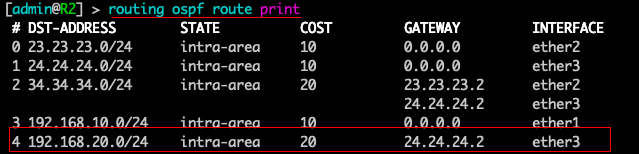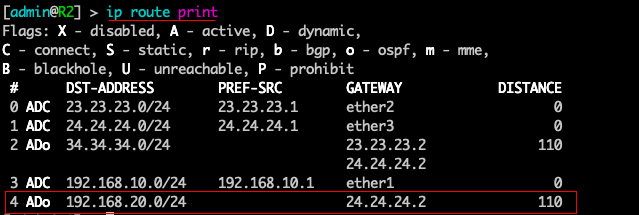As the shown above, network for 192.168.20.0/24 it has a network gateway 24.24.24.2 that is R2-R4.

Now Configure loadbalancing ospf on R2. We will increase cost value for R2-R4 so that it will have same cost value with line of R2-R3-R4.

Add interface on R2 that connect with R4 (ether3), and add new cost value

Now Check again the route information on R2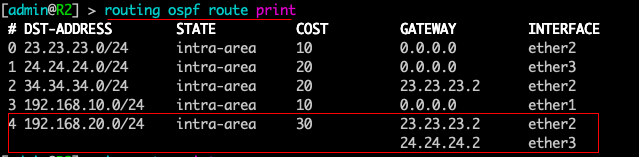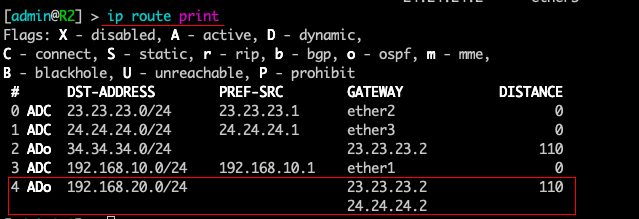As you can see, the network line to 192.168.20.0/24 has a 2 gateway address. the configuration was successfull.

z